# Tetris AI, Experiments 1 & 2: Single Parent Evolutionary Algorithm

13 Nov 2017## Dan Cohen

See author's bio and posts

## Abstract (why not make it formal)

In this experiment, I implement an evolutionary algorithm with no crossover to evolve a neural network with the intention of having it learn to play tetris. I assess the results of my evolutionary algorithm applied to learning tetris. The results show the impact of a high occurrence of mutations(χ) and a high standard deviation for mutations(σ).

Source code is available on github

## Introduction

### Why?

I have been experimenting with artificial intelligence. I was looking for a game. Ideally something with visual output so that I could see the results of my experiments visually. I was inspired to do this by a series of videos I found on youtube about using an evolutionary algorithm to train simple walkers available here. I have previously looked into AI here and here; this is the first time I've seen any notable results.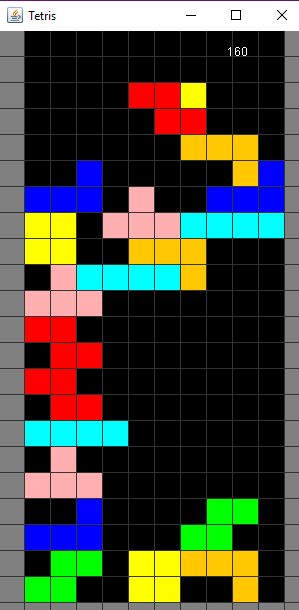### Theoretical Background

#### Neural Network

Neural networks are used as an analogy for the decision making pathways in a brain.A network is structured in layers. Typically, an input layer, any number of hidden layer and an output layer. The advantage of having multiple layers is that higher order features can be understood by the network. For example, if we consider a neural network for analysing images: The first hidden layer can combine the raw data and perhaps understand that a straight line or a corner is a feature in an image. The second hidden layer may be able to combine two straight lines and understand that parallel lines are a feature or combine a straight line and a corner and understand hockey-stick shapes.

My network has three layers. An input layer, a hidden layer and an output layer. This architecture was chosen for its simplicity, since this is the first experiment. Although it may be beneficial to incorporate a more complex architecture in a future experiment.

In a fully connected neural network, such as the one I implemented, every node in a layer is connected to every node in the following layer. Each node holds a value which is calculated from the previous layer.

The values for the input layer are provided to the network by the game.

The value for each node in the hidden layer is calculated using the following formula: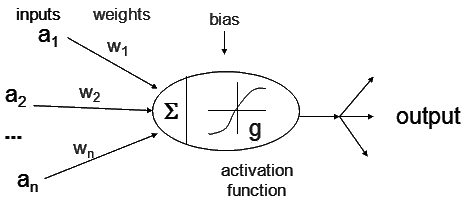In English, you take each node in the input layer and multiply them by their weight. You add all of those together and then add a bias. Then you pass that value through the activation function and that is the value of your node.

You calculate the value of each node in the hidden layer in the same way - and then you do the same for the output layer but using the hidden layer as input.

The output layer is read in order to provide the output result of the neural network. Neural networks have different purposes. They are often trying to solve a classification or a regression problem.

### Regression problem

A regression problem is typically the first you will learn to solve as the simple examples are very easy to understand.

If a network is trying to solve a regression problem, that means it is trying to approximate an unknown function. e.g.

``````f(x) => x^2 + 3x + 2
``````

The weights and biases in the network will typically be adjusted using supervised learning and a training set (a set of values with known input and output) and eventually, the network will be a close approximation to the unknown original function. This is a powerful technique but is not used in this experiment.

Regression problems that I have encountered only have 1 output node and that value is the approximation of the output value of the function. There may be more complex architectures but I am not aware of them.

### Classification problem

A network attempting to solve a classification problem has to answer a question. Is this a cat or a dog? Is this person a risk for diabetes? Is the best move in this situation to turn left or turn right?

This is the goal of my network. Given a particular game state, what is the optimal move?

A classification problem will have one output node for each possible classification. Each of them will have a value between 0 and 1. 0 is translated to "I am absolutely certain it is not this one" and 1 is translated to "I am absolutely certain it is this one".

In order to find out which one your network thinks it is, you just take the highest value from the nodes in the output layer and that is your classification.

### Activation Function

The activation function is a function used to see whether a node has been "activated" or not. It takes into account all nodes connected from the previous layer, all their weights and the bias.

I'm using a sigmoid function for my activation function (here). A sigmoid function is bounded between 0 and 1 and is non-linear. This created a "decision boundary" for the node. More information is available here.

### Weights and Biases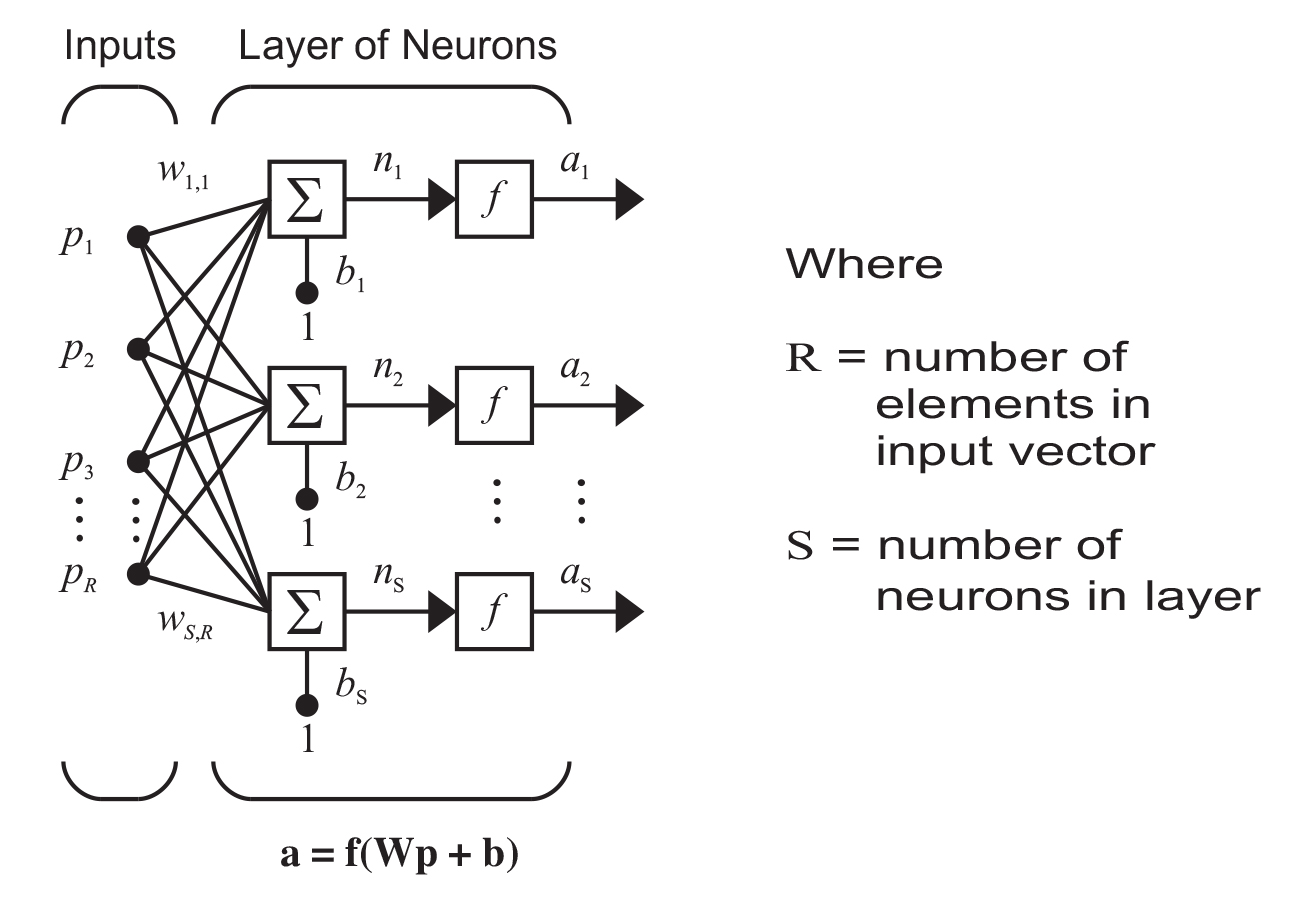Each connection in a neural network has a weight. It is factored in to the calculation of the value of the node it is linking to. Each node, not including the input layer, has a bias. The initial weights and biases are usually randomly assigned. This gives a good place for the network to start.

The network is defined by the structure of the layers, the connections between them and their weights and biases. The structure and connections remain constant throughout an experiment but the weights and the biases will change.

When you talk about "training a neural network" you are talking about modifying the weights and biases to produce better results. They are the key.

### Evolutionary Algorithm

In my experiment, I used an evolutionary algorithm to train the network. the evolutionary algorithm (EA) is not the most efficient, but it is easy to conceptualise. That's why I chose it.

The fundamental principle of EA is generations. Each generation is a group of networks that are all different. You allow each network to play through a game of tetris and then you eliminate the worst of them. The surviving population is allowed to reproduce. The idea is that the surviving networks are more likely to perform better.

Of course, it wouldn't be evolutionary without evolution. So there must be mutations. This is how EA allows networks to progress and perform better and better.

My implementation of EA is unusual in that each network has only one parent. The parent network is duplicated and passed through a series of mutations. The child network has a chance of performing better or worse than the parent. Because the worst performers are eliminated, the average performance of the population increases over time as the positive changes to the networks are compounded.

It's very common for EA to make use of a crossover algorithm. which allows a child to have multiple parents. It is a way of allowing features developed in one network to be combined with features developed in another network. This is the real magic of EA. Unfortunately I was not aware of it at the start of my experiment.

### Mutation

A mutation is a randomly occurring change to a weight or bias. I am using a gaussian distribution (otherwise known as a normal distribution) to achieve a situation where most of the mutations are within a smaller limit, but a small number of mutations are much larger.

The formula I'm using is:

``````w = w + (w * r)
``````

where `w` = weight and `r` = a random number from a guassian distribution, centered at 0. code

This means that 68% of the time, the weight will change by less than σ (plus or minus), 95% will change by less than 2*σ (plus or minus) and 99.7% of the time will change by less than 3*σ

Here is a more detailed explanation of standard deviation.

### Fitness Function

A key point of EA is evaluating the performance of a network. A fitness function is used to provide a numeral value for the performance of a network. The fitness function I use is described further down.

### The Game

Tetris is... well known... but the rules I use are worth formalising as they are not a perfect representation of the gameplay.

• Play Area: The play area in tetris is 10x20. There are 2 blocks above the top row so that the pieces are never out of view.
• Tetrominos: "Tetromino" is the name for the blocks that fall from the top. Each tetromino is a configuration of 4 blocks. They come in 7 varieties (I, J, L, T, S, Z, O) corresponding to their shape.
• Well: The well is the fixed blocks in the game. A tetromino is fixed to the well when it lands on the bottom of the play area or another tetromino.
• Pacing: Every frame, the current tetromino drops down by one block due to "gravity".
• Controls: The network has the opportunity to make one move per frame. This is done in addition to the gravity drop. The following 7 moves are legal:
• move left
• move right
• rotate clockwise
• rotate counter-clockwise
• drop by one
• drop to bottom
• no action
• Scoring: Points are scored when a line is cleared. If multiple lines are cleared at once, the reward is greater.
• 1 line -> 10,000 points
• 2 lines -> 30,000 points
• 3 lines -> 50,000 points
• 4 lines -> 80,000 points

## Methodology

### Neural Network Architecture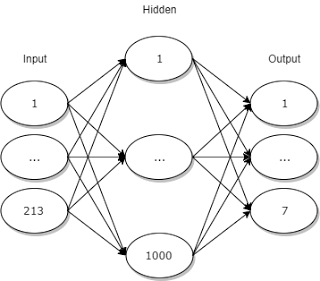#### Input Layer

The input layer has 213 nodes. They can be separated into 4 segments

1 -> 200: the squares of the play area. The value of each is either 1 if there is a block in there or 0 if there is not.

201 -> 207: these represent which tetromino is currently in play. They are all zero except the one which matches the correct tetromino. e.g. if it is a 'T' tetromino, the values of these nodes may look like this: {0, 0, 0, 1, 0, 0, 0}

208 -> 209: The x and y co-ordinates of the tetromino

210 -> 213: The rotation of the tetromino. The tetromino can exist in 1 of four rotations. So I use four nodes to represent that. e.g. if the tetromino is upside-down, the values may look like this {0, 1, 0, 0}

#### Hidden Layer

The hidden layer has 1000 nodes. This number was chosen by rule of thumb. I'm sure it could be made smaller with minimal negative impact.

#### Output layer

The output layer has seven nodes. Each node represents a different move that the neural network can choose. The node with the largest resultant value is chosen.

### Initialisation of the weights and biases

The random values are selected using Random.nextGaussian()).

For the weights, I divide the random gaussian by the size of the layer it is in.

For the biases, I divide the random gaussian by 10000.

These balancing constants were discovered through trial and error.

### Fitness Function

The most naive fitness function is simply the score of the bot in the game.

In my first iteration of EA, that is exactly what I did. I found it to not be enough information to guide the networks to any positive results. The fitness function I have ended up with is augmented with an analysis on the amount of empty space per row. The implementation of this is here

This augmentation can add roughly ±1000. As such, the score from clearing lines will be the dominant factor in the fitness function. However if the network is unable to clear any lines, this fitness function encourages the evolution of networks that will fill space.

## Generations

Each generation has 1000 individuals. Each individual plays a single game of tetris.

#### Formation of next generation

The worst performing 500 networks are discarded. Each of the remaining 500 is preserved into the next generation and each of the remaining 500 produces a single child. The child is mutated at random.

Each weight and bias in the child has a configurable chance of mutating(χ). The mutations themselves exist in a normal distribution with configurable standard deviation(σ) and mean(μ). I conducted two experiments varying χ and σ.

## Results

### Experiment 1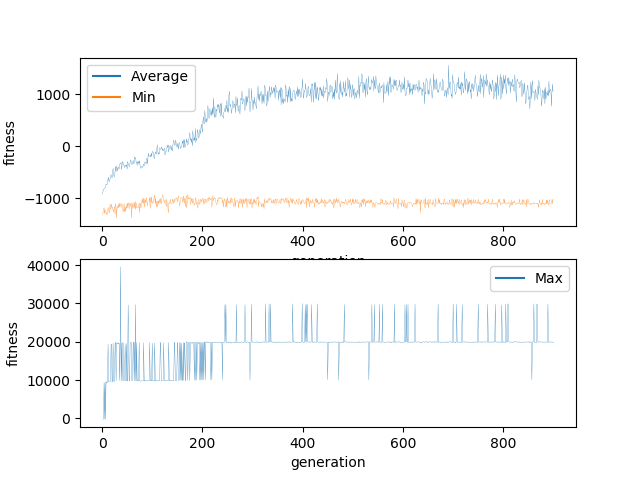For this experiment, the mutation parameters were:

χ ≅ 0.4
σ = 1
μ = 0

The high χ means that every time a child is generated, roughly 40% of the weights and biases will be mutated. The high σ means that every weight or bias which is mutated has a 32% chance to change by more than 100% in either direction.

This means that each child is 40% different from its parent and 13% of the weights and biases are more than 100% different. This is probably too much to allow for consistent evolution. As you can see, the minimum never increased beyond -1000. This suggests me that even though the worst performers were culled in every generation, new worst performers were generated every generation. When watching visually, I observed a significant number of individuals which were not making any moves and just stacking pieces on top of each other.

Regardless, the networks did successfully evolve. Evidenced by the average climbing somewhat consistently, although it appears to have gotten stuck in a local minima. The highest score ever achieved was 40,000. This means that networks able to clear 4 lines were generated but no network was ever generated which was able to actively seek out or create situations in which it could increase its score. In 900 generations I would have expected far more progress.

### Experiment 2For this experiment, the mutation parameters were:

χ = 0.1
σ = 1/3
μ = 0

This experiment exhibits much of the same characteristics of the initial experiment. This experiment also appears to have gotten stuck in a local minima.

My conclusion is that while the mutation parameters may have an impact, they are not the reason the experiment has failed.

## Conclusion

This experiment has shown that the mutation parameters have a fairly minor impact on EA performance.

I believe there are three avenues within EA for dramatic improvement of performance:

1. Implement a crossover function so that features may be shared between networks
2. Don't just take the best 500 - allow a chance for worse performing networks to survive to allow the solution to escape local minima
3. Abandon the fully connected neural network and implement a more complex architecture

New blog post to follow with results of the next experiment.

## References

https://en.wikipedia.org/wiki/Artificial_neural_network

https://en.wikipedia.org/wiki/Genetic_algorithm

original source of tetris engine

This post was cross-posted to hacking dan.